Dependent Sources and Op Amps
More circuit elements

 Outline: Dependent Sources Op Amps Behavior of Op Amps with Negative Feedback Basic Op Amp Circuits More analysis of Op Amp and Dependent Source Circuits

Dependent Sources

A Dependent Source is similar to an independent source except its value
depends on some other voltage or current elsewhere in the circuit.

A dependent source represents a device that amplifies or responds to another
signal elsewhere in the circuit.

In the lab we will build amplifier circuits using Op Amps (Operational Amplifiers).

There are four types of Dependent Sources:

Voltage Controlled Voltage Source (VCVS) - This is a voltage source that depends on some voltage.
Current Controlled Voltage Source (CCVS) - This is a voltage source that depends on some current.
Voltage Controlled Current Source (VCCS) - This is a current source that depends on some voltage.
Current Controlled Current Source (CCCS) - This is a current source that depends on some current.

### This is a VCVS:

This is a dependent voltage source. The voltage across the
source depends on Vx.

For example, if Vx=5V and u=3 then the voltage across the
source is uVx or 15V.

The value of u is unitless and is determined by how the dependent
source is designed.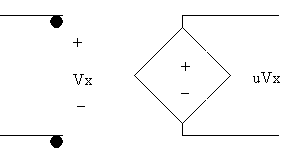### This is a CCVS:

This too is a dependent voltage source. The voltage across the
source depends on the current Ix.

For example, if Ix=2A and u=10 then the voltage across the
source is uIx or 20V.

The value of u is determined by how the dependent source is designed.

### Since the dependent source puts out voltage, its value is always expressed in volts, even though the source depends on a current. u converts from Amps to Volts.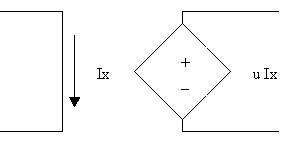### This is a VCCS:

This is a dependent current source. The current through the
source depends on the voltage Vx.

For example, if Vx=10V and g=0.5 then the current through the
source is gIx or 5A.

The value of g is determined by how the dependent source is designed.

### Since the dependent source puts out current, its value is always expressed in amps, even though the source depends on a voltage. g converts from Volts to Amps.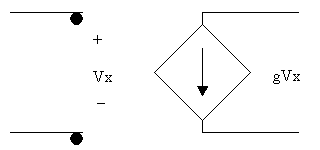### This is a CCCS:

This is a dependent current source. The current through the
source depends on Ix.

For example, if Ix=3A and B=2 then the current through the
source is BIx or 6A.

The value of B is unitless and is determined by how the dependent
source is designed.## Study Problems

After clicking on the following link enter 4-1 for the problem and 1 for the step:
Study Problem 4-1
After clicking on the following link enter 4-2 for the problem and 1 for the step:
Study Problem 4-2

Top of Page

Op Amps

Op Amp stands for Operational Amplifier

Op amps are used in circuits which respond to or amplify a signal.
We can construct a dependent source in the lab using an Op Amp circuit.

An Op Amp is a three terminal device on paper:Where Vin = (V+ - V-)

An Op Amp is an integrated circuit with many connections to it along with power
required to energize it. However 'on paper' we will treat it as a simple three terminal
device that exhibits the following behavior:
• Very high open loop gain. In other words when there is no
feed back the gain/amplification of the Op Amp is very high, on
the order of 10,000 to 100,000.
We will talk about feedback later.

• Very high input resistance, so high that we will assume that
no current can enter through either V+ or V-.

Therefore an Op Amp can be substitued with the 'Ideal Voltage Amp Model':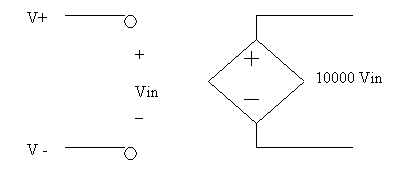In the above model the op amp gain is 10000

Summary:

We can create dependent sources with the Op Amp device.
If there is no feed back, (or loop connecting the output to the input)
then we can replace the Op Amp with the Idea Voltage Amp Model shown above.

 This a simple Op Amp circuit: Using the Ideal op amp model, find Vout. The first step is to replace the Op Amp with the model above.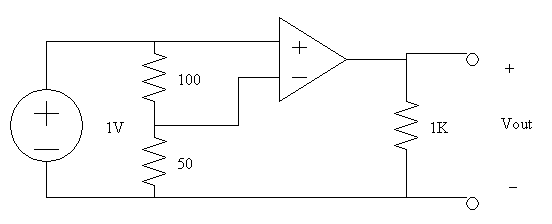This is the same circuit with the Op Amp replaced with the Ideal Model: We now will do analysis using KVL/KCL and Ohm's Laws to find Vout. By inspection we can see that Vout = 10000(Vin), therefore all we need to find is Vin. Also by inspection we see that V+ = 1V because V+ is connected to the source. The 100 and 50 Ohm resistors are in series because they carry the same current (note that no current goes into the op amp at V+ or V-). Applying Voltage Division V50 = 1V[50/(50+100)] V50 = 1V(1/3) = 0.333V V- = V50 = 0.333V Vin = (V+ - V-) = 1V - 0.333V = 0.67V Vout = 10000Vin = 10000*(0.67V) = 6700V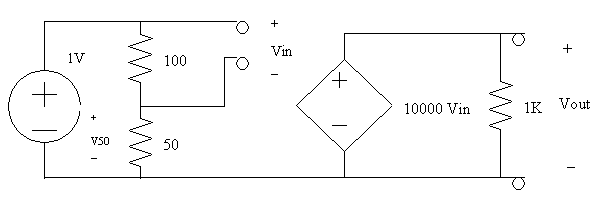Top of Page

Behavior of Op Amps with Negative Feedback

When op amp circuits contain negative feedback a simpler model can be used
to analyze the circuit (other than the Ideal Op Amp Model shown above).

But first, what is Feedback?
Feedback is when output information is fed back to the input.
The purpose of feedback is to create a more stable system.

What is Positive Feedback?
When output information is fed back to the positive input V+.
This leads to an unstable system - one that is out of control.

Example of a Positive Feedback System:
When you put a microphone up to a speaker the small but significant buzz coming out
of the speaker goes into the microphone, gets amplified and then comes out of the speaker.
This slightly louder buzz then in turn goes into the microphone, gets amplified and again comes
out of the speaker a little louder than before. This cycle goes on indefinitely until you
hear very loud, painful ringing/screeching coming out of the speaker.

What is Negative Feedback?
When output information is fed back to the negative input V-.
This leads to a stable system. One where the output remains
constant.

Example of a Negative Feedback System:
When you feel hungry messages are sent to your brain telling you that it is time to eat.
As you eat and your stomach fills up, messages go to your brain this time telling you that
you are not hungry. Thus you stop eating. These messages to the brain are a negative
feedback system, keeping you nourished, not over or under-fed.

Here is an op amp circuit with negative feedback:

We will first analyze this circuit using the Ideal Voltage Op Amp Model
shown in the last section.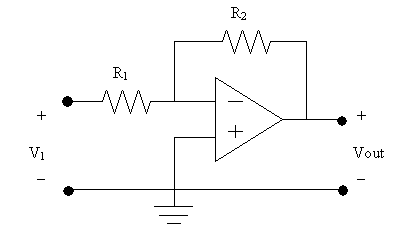Here is the same circuit with the Op Amp replaced
with the Ideal Model:

Now we will perform analysis -

KVL on the outside: (Note that the current in the two resistors is the same)
-V1 + iR1 + iR2 + Vout = 0
Vout = V1 - i(R1 + R2)

Now we will do KVL starting at Vin going clockwise:
+Vin + iR2 + AVin = 0
Solving for i: i = [-Vin(1 + A)]/R2
Since A = 10000 or more, then A+1=A
Therefore i = [-Vin*A]/R2

Now we plug i into the first equation:
Vout = V1 - (-Vin*A/R2)(R1 + R2)
Vout = V1 + (Vin*A)(R1/R2 + 1)

By Inspection we know AVin = Vout
Vout = V1 + (Vout)(R1/R2 + 1)
Vout = V1 + Vout*R1/R2 + Vout
Vout - Vout - Vout*R1/R2 = V1 (The first two terms cancel out)
Vout = -(R2/R1)*V1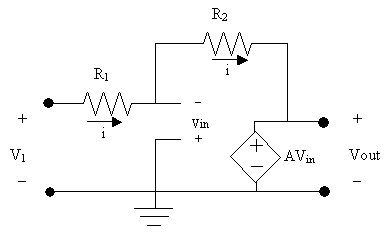### Note that Vout does not depend on A. Due to negative feedback, if A approaches infinity, Vout still remains constant. Therefore we can go ahead and assume that A is infinity. But if we assume this then Vin must be very, very close to zero. i.e. (V+ - V-) = 0 In summary we can assume the following about our op amp model when there is negative feedback: (V+ - V-) = 0, in other words V+ = V- This means there is a virtual short between the two inputs. No current goes into the op amp

Below we show this new op amp model!

Summary
When an op amp circuit contains negative feedback (i.e. some type of circuit
connection back to V-), then the simple model shown below can be used.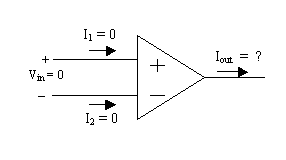With this model assume during analysis that current into V+ and V- is always zero
and assume that V+ = V- (or Vin = 0).
Never apply KCL around the op amp. If you did this iout would be zero, which it may or may not be.
There are other connections to the op amp, such as power, which are not shown on paper.

## Therefore, never apply KCL around the op amp.

Top of Page

Basic Op Amp Circuits

In this section we will analyze some common op amp circuits
which have negative feedback. Therefore we will use the op amp
model introduced in the last section.

This circuit was analyzed in the last section using the Ideal Op Amp Model.
Now we will analyze it with the other op amp model:

First we assume that V+ = V- (i.e. Vin = 0) and that I1 = 0 and I1 = 0

Since I1 = 0 then IR1 = IR2
By Inspection V- is connected to the ground node, therefore V- = 0
Since V+ = V- then V+ = 0

KVL loop equation 1:
-V1 + IR1R1 + Vin = 0
V1 = IR1R1
IR1 = V1/R1

KVL loop equation 2:
- Vin + IR2R2 + Vout = 0
But recall that IR1 = IR2, therefore IR2 = V1/R1
Plugging IR2 into the equation gives:
- Vin + (V1/R1)R2 + Vout = 0

Recall that Vin = 0, therefore the equation reduces to:
(V1/R1)R2 + Vout = 0

### Vout = - V1(R2/R1) This circuit is called an Inverting Amplifier because the output is inverted compared to the input V1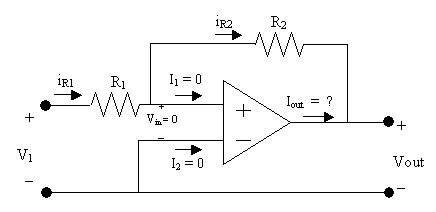Here we will analyze the circuit to find Vout/V1:

Since I2 = 0 then i through R1 equals i through R2

KVL loop equation 1:
-V1 + Vin + iR1 = 0
Recall that Vin = 0
Therefore V1 = iR1
i = V1/R1

KVL loop equation 2:
-iR1 - iR2 + Vout = 0
-i(R1 + R2) + Vout = 0
Vout = i(R1 + R2)
Now we can plug in i = V1/R1 to get:
Vout = V1/R1(R1 + R2)

### Vout = V1(1 + R2/R1) This circuit is called a Noninverting Amplifier because the output is amplified but it is not inverted compared to the input.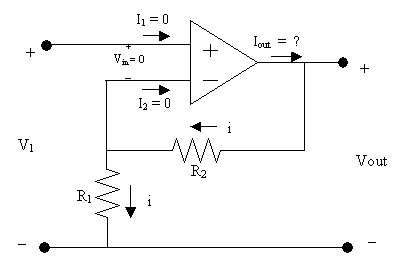Here we will analyze the circuit to find Vout/V1:

Here i = 0 because I2 = 0
V+ = V1 because they are the same node
Since V+ = V-, the V- = V1
But Vout = V- because they are the same node
Therefore Vout = V- = V1

### Vout = V1 This circuit is called a voltage follower or buffer. The output equals the input. The input is buffered from the output. We will see in the next section why this is a useful circuit.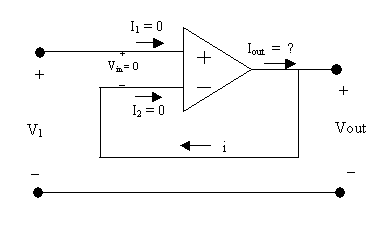Summary

These standard circuits can be used as building blocks in more complicated
circuits. You can apply the above formulas to determine their output.

Top of Page

More analysis of Op Amp and Dependent Source Circuits

The following study problems reinforce this section on Dependent Sources
and op amps:

## Study Problems

After clicking on the following link enter 4-3 for the problem and 1 for the step:
Study Problem 4-3
After clicking on the following link enter 4-4 for the problem and 1 for the step:
Study Problem 4-4
After clicking on the following link enter 4-5 for the problem and 1 for the step:
Study Problem 4-5

Top of Page

Back To Index